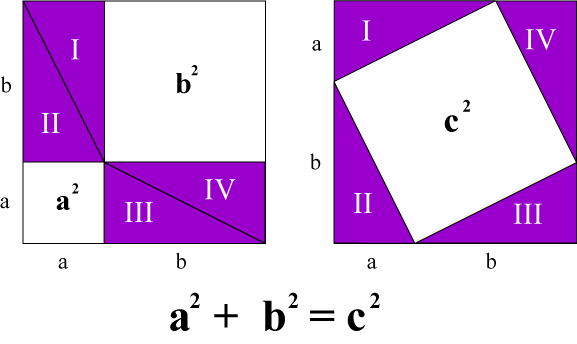## A Picture Proof of the Pythagorean TheoremBoth squares have sides of length a + b and therefore both have the same area.

The purple region in each square consists of four abc right triangles, and so both purple regions have the same area.

We conclude that the white regions in the two squares have equal areas, and...

QED

This image was taken from a T-shirt conceived by Sen Nagata and designed by me for the Amherst College Student Chapter of the Mathematical Association of America.

Many More Picture Proofs of the Pythagorean Theorem